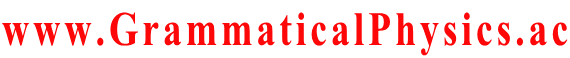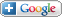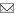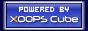Welcome Guest
www.GrammaticalPhysics.ac

Access Detector
3 user(s) are online (2 user(s) are browsing Forum)

Members: 1
Guests: 2

SourceCodeOf_HumanGenome more...

# Discretized Classical Model of the New Equation

SourceCodeOf_HumanGenome > Discretized Classical Model of the New Equation @ 2014/5/13 17:31
In this post, I try to make n=∞ straight time version of Cartoon of the Equation.

Below I solve classically the equation of motion of the mechanical system defined by the following Hamiltonian.
H(p; x) = Qφ(x) + [1／(2M)] Σk=－∞ [pk － QAk(x)]2.
M = mεα2, Q = m.
Ak(x) = α(xk+1 － xk), φ(x) = －[1／(2ε)] Σk=－∞ (xk+1 － xk)2.
Derivation of this Hamiltonian is the same as one of Cartoon of the Equation.

Hamilton's canonical equations of motion of this system is as follows.
dxj／dt = ∂H／∂pj = (pj － QAj)／M,
dpj／dt = －∂H／∂xj = (Q／M)Σk (pk － QAk)∂Ak／∂xj － Q∂φ／∂xj.

From the first of these equations, it is derived that
pj = Mdxj／dt + QAj.
Substituting this into the second of the equations, it is derived that
M(d／dt)2 xj + QdAj／dt
= (Q／M)Σk (pk － QAk)∂Ak／∂xj － Q∂φ／∂xj
= (Q／M)Σk (Mdxk／dt)∂Ak／∂xj － Q∂φ／∂xj.
For the term dAj／dt in the left hand side of this equation, substituting that
dAj／dt = Σk (∂Aj／∂xk)dxk／dt,
it is derived that
M(d／dt)2 xj = (Q／M)Σk (Mdxk／dt)∂Ak／∂xj － QΣk (∂Aj／∂xk)dxk／dt － Q∂φ／∂xj.
∴ M(d／dt)2 xj = QΣk (dxk／dt)(∂Ak／∂xj －∂Aj／∂xk) － Q∂φ／∂xj.

Here I use the concrete expressions of A and φ.
∂Ak／∂xj = α(δk+1,j － δk,j), ∂Aj／∂xk = α(δj+1,k － δj,k) = α(δk-1,j － δk,j)
∴ ∂Ak／∂xj － ∂Aj／∂xk = α(δk+1,j － δk-1,j) = α(δj-1,k － δj+1,k).
∂φ／∂xj = －(1／ε)[(xj － xj-1) － (xj+1 － xj)] = －(2／ε)[xj － (1／2)(xj-1 + xj+1)].

Substituting these expressions into the above result, we get the concrete form of the equation of motion of the main problem of this post as follows.
M(d／dt)2 xj = Qα(dxj-1／dt － dxj+1／dt) + Q(2／ε)[xj － (1/2)(xj-1 + xj+1)].
Substituting mεα2 and m for M and Q in this equation, we get the following expression as the final form of the equation of motion.
(d／dt)2 xj = [1／(εα)](dxj-1／dt － dxj+1／dt) + [2／(ε2α2)][xj － (1/2)(xj-1 + xj+1)].

This equation is the opposite of the following equation representing, as it were, an automatic collision avoidance system.
M(d／dt)2 xj = Qα[(dxj-1／dt － dxj／dt) + (dxj+1／dt － dxj／dt)] － Q(2／ε)[xj － (1/2)(xj-1 + xj+1)].

---
---

Multiplying both sides of the equation by dxj／dt and summing over j, we get the following equation representing the energy conservation law.
(d／dt)Σa≦j≦b(M／2)(dxj／dt)2 = Qα[(dxa-1／dt)(dxa／dt) － (dxb／dt)(dxb+1／dt)] + (Q／ε)(d／dt)Σa≦j≦b[(xj)2 － xjxj+1] － (Q／ε)(xa-1 dxa／dt － xb dxb+1／dt).
∴ (d／dt)Σa≦j≦b[(M／2)(dxj／dt)2 － (Q／ε)xj(xj － xj+1)]
= Qα[(dxa-1／dt)(dxa／dt) － (dxb／dt)(dxb+1／dt)] － (Q／ε)(xa-1 dxa／dt － xb dxb+1／dt).
∴ (d／dt)Σa≦j≦b[(M／2)(dxj／dt)2 － (Q／ε)xj(xj － xj+1)]
= Q[α(dxa-1／dt)(dxa／dt) － (1／ε)xa-1(dxa／dt)] － Q[α(dxb／dt)(dxb+1／dt) － (1／ε)xb(dxb+1／dt)].

The right hand side of this equation represents the time derivative of energy possessed by the part such that a≦j≦b, and the left hand side of this equation represents
(energy flow at j=a) - (energy flow at j=b).
Therefore this equation represents the energy conservation law.

---
---

To investigate the character of this problem briefly, now I omit the scalar potential term and try solving the following equation.
(d／dt)2 xj = [1／(εα)](dxj-1／dt － dxj+1／dt).
Introducing a Matrix A defined as
Ajk = δj-1,k － δj+1,k,
the equation is rewritten as
(d／dt)2 xj = [1／(εα)]Σk Ajk dxk／dt.

Then the solution is writen as follows.
dx／dt = a exp[tA/(εα)] v,
x = aεα exp[tA／(εα)] u + w,
where a is a constant real number, and u, v, w are infinite dimensional constant real column vectors such that v = Au.

---
---

From the point of view of energy conservation, velocity does not increase or decrease as a whole.
This situation is expected to be represented well by
dx／dt = a exp(it × somematrix) v,
but the above solution does not include the imaginary unit at all.
Perhaps this superficial contradiction is cancelled by the fact that the matrix A has a property analogous to the property of a pure imaginary number.
As for the case of a 2×2 matrix, the matrix A defined by the equation:
Ajk = δj-1,k - δj+1,k
is actually a representation of the imaginary unit.
We can fully express this understanding by redefining the matrix A' as A'jk = －iAjk and rewriting as
dx／dt = a exp[itA'／(εα)] v.
Here A' is Hermitian.
We know well from quantum mechanics that this represents a rotation in an infinite dimensional space.

---
---

If A-1 exists, u = A-1 v.
However, perhaps A-1 does not exist, because there is an invariant vector under the transformation exp[tA／(εα)] so zero is an eigenvalue of A so the determinant of A is zero.
This is understood by considering about A' = -iA.
A' is Hermitian so it is diagonalized to the form whose all diagonal elements are eigenvalues of A' so the determinant of A' is the product of all its eigenvalues.
Therefore, if zero is an eigenvalue of A' then the determinant of A' is zero.
The eigenvalues of A are completely same as the eigenvalues of A'.
The determinant of A is different from one of A' only by a factor, so if zero is an eigenvalue of A then the determinant of A is zero.
It is shown below that there is an invariant vector under the transformation exp[tA／(εα)].

The space of all classical solutions of the main equation of this post which is an equation of motion is
{ x | ∃ξ,η; ∀j; xj = jξ + η }.
Every vector in this space is written in the form:
a ∂x/∂ξ + b ∂x/∂η.
The j-th component of this vector is
(a ∂x/∂ξ + b ∂x/∂η)j = aj + b.

This individual vector is not necessarily invariant under exp[tA/(εα)], but the space which consists of all such vectors is invariant under exp[tA/(εα)].
I prove it below.

[A(a ∂x/∂ξ + b ∂x/∂η)]j
= Σk Ajk (a ∂x/∂ξ + b ∂x/∂η)k
= Σkj-1,k - δj+1,k)(ak + b)
= [(j-1)a + b] - [(j+1)a + b]
= -2a.
(So, the vector is invariant if a = 0.)

[A2 (a ∂x/∂ξ + b ∂x/∂η)]j
= Σk Ajk (-2a)
= Σkj-1,k - δj+1,k)(-2a)
= 0.

An (a ∂x/∂ξ + b ∂x/∂η) = An-2 A2 (a ∂x/∂ξ + b ∂x/∂η) = An-2 0 = 0 (n≧3).

{exp[tA/(εα)](a ∂x/∂ξ + b ∂x/∂η)}j
= {[1 + tA/(εα)](a ∂x/∂ξ + b ∂x/∂η)}j
= (aj + b) + [t/(εα)](-2a)
= aj + [b - 2at/(εα)].

∴ exp[tA/(εα)](a ∂x/∂ξ + b ∂x/∂η)
= a ∂x/∂ξ + [b - 2at/(εα)]∂x/∂η
∈ the original space.

This justifies our thinking of exp[tA/(εα)] as a rotation around the space of all classical solutions as an invariant axis.

---
---

To write down exp[tA/(εα)] explicitly, below I try to get explicit expressions of all powers of A.

(A2)jk
= ΣsAj,sAs,k
= Σsj-1,s - δj+1,s)(δs-1,k - δs+1,k)
= δj-2,k - 2δj,k + δj+2,k

(A3)jk
= Σsj-2,s - 2δj,s + δj+2,s)(δs-1,k - δs+1,k)
= δj-3,k - 3δj-1,k + 3δj+1,k - δj+3,k

(A4)jk
= Σsj-3,s - 3δj-1,s + 3δj+1,s - δj+3,s)(δs-1,k - δs+1,k)
= δj-4,k - 4δj-2,k + 6δj,k - 4δj+2,k + δj+4,k

(A5)jk
= Σsj-4,s - 4δj-2,s + 6δj,s - 4δj+2,s + δj+4,s)(δs-1,k - δs+1,k)
= δj-5,k - 5δj-3,k + 10δj-1,k - 10δj+1,k + 5δj+3,k - δj+5,k

(An)jk
= Σ0≦s≦n/2(-1)sc1(s,n)δj-n+2s,k + Σ0≦s＜n/2(-1)n+sc2(s,n)δj+n-2s,k,
cr(s,n+1) = cr(s,n) + cr(s-1,n),
cr(0,n) = 1.

Using these results in the expression:
dx／dt = a exp[tA/(εα)] v,
it is derived that
dxj／dt = Σn=0 (1/n!)[t／(εα)]n0≦s≦n/2 (-1)s c1(s,n) δj-n+2s,0 - Σ0≦s＜n/2 (-1)s c2(s,n) δj+n-2s,0]
in the case that a = 1 and vj = δj0.

I can not go further because the recurrence relation for c can not be solved fully.
It seems to be impossible to write down fully c1(s,n) and c2(s,n) as functions of s and n.

---
---

To take the scalar potential term into account, I rewrite the main equation of this post to the following form.
(d／dt + F)(d／dt + G)x = 0.
By comparing this expression with the original equation, the condition to determine F and G is found to be as follows.
(F + G)jk = [1／(εα)](δj+1,k － δj－1,k),
(FG)jk = [1／(ε2α2)](δj－1,k + δj+1,k － 2δjk).

Then the solution is written as follows.
(d／dt + G)x = b exp(－tF) v1,
x = exp(－tG)[v1 + b ∫ctdt' exp(t'G)exp(－t'F)v2].

Below I try to know F and G concretely.

Fjk = Σs=－∞ fs δj+s,k,
Gjk = Σs=－∞ gs δj+s,k.

(FG)jk = Σr Fjr Grk
= Σr,s1,s2 fs1 δj+s1,r gs2 δr+s2,k
= Σs1,s2 fs1 gs2 δj+s1+s2,k

The condition for FG means that (FG)jk = 0 if |j－k| ≧ 2.
So f and g must satisfy the following condition.
|r| ≧ 2 ⇒ Σs1+s2=r fs1 gs2 = 0,
∴ |r| ≧ 2 ⇒ Σs fs gr－s = 0.
The condition for FG means that (FG)jk = －2／(ε2α2) if j = k.
So f and g must satisfy the condition:
Σs fs g－s = －2／(ε2α2)
derived as the case that r = 0.
The condition for FG means that (FG)jk = 1／(ε2α2) if j+1 = k.
So f and g must satisfy the condition:
Σs fs g1－s = 1／(ε2α2)
derived as the case that r = 1.
The condition for FG means that (FG)jk = 1／(ε2α2) if j－1 = k.
So f and g must satisfy the condition:
Σs fs g－1－s = 1／(ε2α2)
derived as the case that r = －1.

From the condition for F + G, the following conditions for f and g are derived.
f1 + g1 = 1／(εα),
f－1 + g－1 = －1／(εα),
not(s = 1 or s = －1) ⇒ fs + gs = 0.

Using the condition derived from the condition for F + G, now I eliminate f in the condition derived from the condition for FG.

|r| ≧ 2 ⇒ f1 gr－1 + f－1 gr+1 + Σ1≠s≠－1 fs gr－s = 0.
∴ |r| ≧ 2 ⇒ [1／(εα) － g1]gr－1 + [－1／(εα) － g－1]gr+1 + Σ1≠s≠－1 (－gs)gr－s = 0.
∴ |r| ≧ 2 ⇒ [1／(εα)]gr－1 － [1／(εα)]gr+1 = Σs gs gr－s.

As for the part as r = 0,
f1 g－1 + f－1 g1 + Σ1≠s≠－1 fs g－s = －2／(ε2α2).
∴ [1／(εα) － g1]g－1 + [－1／(εα) － g－1]g1 + Σ1≠s≠－1 (－gs)g－s = －2／(ε2α2).
∴ [1／(εα)]g－1 － [1／(εα)]g1 + 2／(ε2α2) = Σs gs g－s.

As for the part as r = 1,
f1 g1－1 + f－1 g1+1 + Σ1≠s≠－1 fs g1－s = 1／(ε2α2).
∴ [1／(εα) － g1]g0 + [－1／(εα) － g－1]g2 + Σ1≠s≠－1 (－gs)g1－s = 1／(ε2α2).
∴ [1／(εα)]g0 － [1／(εα)]g2 － 1／(ε2α2) = Σs gs g1-s.

As for the part as r = －1,
f1 g－1－1 + f－1 g－1+1 + Σ1≠s≠－1 fs g－1－s = 1／(ε2α2).
[1／(εα) － g1]g－2 + [－1／(εα) － g－1]g0 + Σ1≠s≠－1 (－gs)g－1－s = 1／(ε2α2).
[1／(εα)]g－2 － [1／(εα)]g0 － 1／(ε2α2) = Σs gs g－1－s.

Therefore, the complete set of conditions for determine g are as follows.
|r| ≧ 2 ⇒ [1／(εα)]gr－1 － [1／(εα)]gr+1 = Σs gs gr－s,
[1／(εα)]g－1 － [1／(εα)]g1 + 2／(ε2α2) = Σs gs g－s,
[1／(εα)]g0 － [1／(εα)]g2 － 1／(ε2α2) = Σs gs g1－s,
[1／(εα)]g－2 － [1／(εα)]g0 － 1／(ε2α2) = Σs gs g－1－s.

After determining g from these conditions, f is determined from the condition derived from the condition for F + G.

The number of equations for determining g is equal to the number of elements of g, and so perhaps the solution of the set of equations exists, but it is too difficult to be solved.

---
---

Though I can not write the exact solution because of such a difficulty, perturbation method saves us from giving up knowing further.

In convenience, here I paste again the main equation to be solved.
(d／dt)2 xj = [1／(εα)](dxj-1／dt － dxj+1／dt) + [1／(ε2α2)](2xj － xj-1 － xj+1).

To apply perturbation method to this equation, I replace the coefficient of the scalar potential part by a new constant k.
(d／dt)2 xj = [1／(εα)](dxj-1／dt － dxj+1／dt) + k(2xj － xj-1 － xj+1).

Then I substitute a power expansion of x in terms of k:
xj = Σn=0 kn xnj
into the equation, and I equate both sides order by order as usual.

Below I try calculating the functions corresponding to the first two orders.
(d／dt)2 x0j = [1／(εα)](dx0j-1／dt － dx0j+1／dt).
(d／dt)2 x1j = [1／(εα)](dx1j-1／dt － dx1j+1／dt) + (2x0j － x0j-1 － x0j+1).

x0 has already been derived above when I omitted the scalar potential term.

From the second equation, it is derived that
(d／dt)2 x1 = [1／(εα)] A dx1／dt + B x0,
where
Ajk = δj-1,k － δj+1,k,
Bjk = 2δjk － δj-1,k － δj+1,k.
Solving this equation, we get the expression for dx1／dt:
dx1／dt = exp[tA／(εα)]∫ctdt' exp[－t'A／(εα)]Bx0(t').
Substituting x0 into this expression, we get the following expression.
dx1／dt = exp[tA／(εα)]∫ctdt' exp[－t'A／(εα)]B{aεα exp[t'A／(εα)] u + w}.

To reduce this expression, here I calculate the commutation relation between A and B.
[A, B]jk = Σs AjsBsk － Σs BjsAsk
= Σsj-1,s － δj+1,s)(2δsk － δs-1,k － δs+1,k) － Σs(2δjs － δj-1,s － δj+1,s)(δs-1,k － δs+1,k)
= (2δj-1,k － δj-2,k － δj,k － 2δj+1,k + δj,k + δj+2,k) － (2δj-1,k － 2δj+1,k － δj-2,k + δj,k － δj,k + δj+2,k)
= 0.

Because of this vanishment,
exp[－t'A／(εα)] B exp[t'A／(εα)]= B,
so the expression for dx1／dt is reduced to the following form.
dx1／dt = exp[tA／(εα)]∫ctdt' {aεαBu + exp[－t'A／(εα)]Bw}
= exp[tA／(εα)]{aεα(t － c)Bu － εα exp[－tA／(εα)]w' + εα exp[－cA／(εα)]w'}
= εα{a(t － c)exp[tA／(εα)]Bu － w' + exp[(t － c)A／(εα)]w'}.
where w' and w are chosen such that Aw' = Bw.

Let's consider the special case such that w = w' = 0 to sketch roughly the effect of scalar potential term.
(d／dt)(x0 + kx1)
= a exp[tA／(εα)]v + kεαa(t － c)exp[tA／(εα)]Bu
= a exp[tA／(εα)][v + kεα(t － c)Bu].
This means that a gradually varying vector, which is a linear function of t, is transformed by rotation with some constant angular velocity.

---
Last edited at 2014/06/09/17:20JST
Site Search
 Grammatical Physics SearchGadgets powered by Google
Site Info
Webmasterswooder